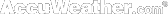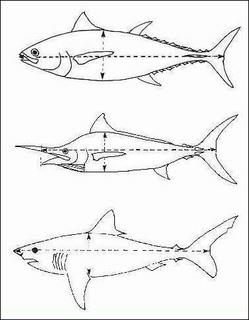## BRUNEI WEATHER FORECAST## Monday, February 25, 2008

### Estimation on Weight of FishThe measurements are all in inches and the final result in pounds, but this can then be transformed into kilograms.

The girth of the fish is measured on the greatest part and then squared.

This number is then multiplied by the body length of the fish, measured as illustrated.

This result is then divided by 800 to get the weight in pounds.

Example:

Girth is 3 feet 4 inches = 40 inches
Body length is 8 feet 4 inches = 100 inches
40 x 40 =1600
1600 x 100 = 160000
160000 / 800 = 200 lbs.

To get the result in kilograms, the final number is then divided by 2.2.

Example:
200 / 2.2 = 88 kg.